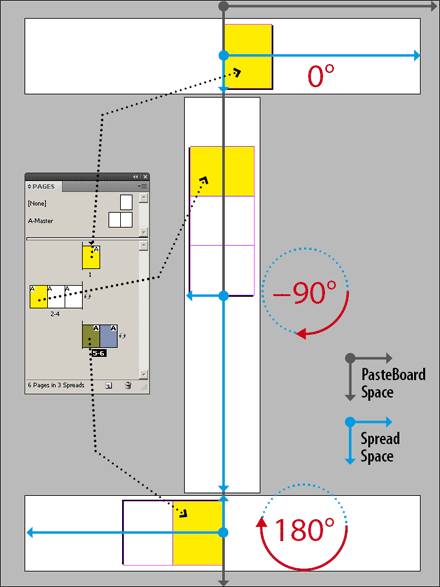And worse, the dark side of rotated spread/page environment is that it significantly impacts the geometry handled by your script. As described in this InDesign Scripting Forum thread you can no longer trust the information provided by `Page.bounds`, or `PageItem.geometricBounds`. You may also have to deal with swapped measurement units, misrepresented object rotation angles, or misleading zero point coordinates.

As a result, a number of existing scripts will have a strange behavior while running in a rotated context —I let you try with Equalizer...

## Rotated View Calculation Script

The least we can do for now is to extend the DOM to provide a method which returns the rotation angle of a `Spread` / `MasterSpread` / `Page`. Harbs offers a clean solution, provided we temporarily allow the script to ‘hit’ the document `viewPreferences` and `zeroPoint` in order to process reliable page bounds. Here is another approach using the power of the `resolve` method (see also “Work Around the Width/Height Gap” ). The trick is very basic: we convert the coordinates of an horizontal vector of the target Spread into the Pasteboard coordinate space, then we calculate the corresponding angle. This method relies on the invariance of the Pasteboard coordinate space:Now to the script:

```Spread.prototype.getRotationAngle =
{
if( !('resolve' in this) ) return 0; // CS3 compat.

var solver = function(pt)
{
return this.resolve(pt,
CoordinateSpaces.PASTEBOARD_COORDINATES);
};

var o = solver.call(this, AnchorPoint.CENTER_ANCHOR);
var m = solver.call(this, AnchorPoint.RIGHT_CENTER_ANCHOR);

return ( m != o )?
( ( m > o )? 0 : 180 ):
( ( m > o )? -90 : 90 );
}

Page.prototype.getRotationAngle = function()
{
return this.parent.getRotationAngle();
}

// sample code
//----------------------------
var myPage = app.activeWindow.activePage;
alert( "Active Page Angle: " + myPage.getRotationAngle() );
```

You can apply the `.getRotationAngle()` method on any `Spread`, `MasterSpread`, or `Page` used in your script. It returns the respective rotation angle in degrees: 0 (no rotation), +90 (counter clock wise rotation), -90 (clock wise rotation), or 180 (turned).